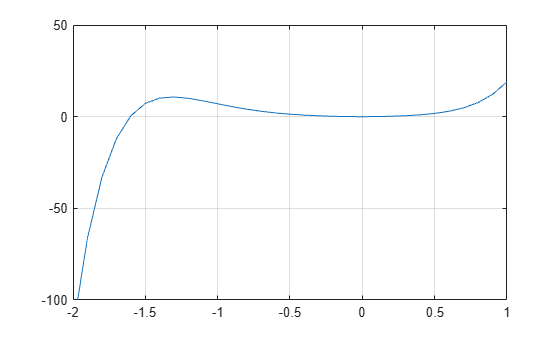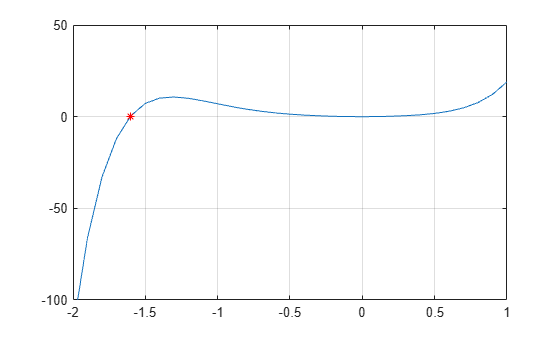Documentation

Roots of Polynomials

This example shows several different methods to calculate the roots of a polynomial.

Numeric Roots

The roots function calculates the roots of a single-variable polynomial represented by a vector of coefficients.

For example, create a vector to represent the polynomial ${x}^{2}-x-6$, then calculate the roots.

p = [1 -1 -6];
r = roots(p)
r =

3
-2

By convention, MATLAB® returns the roots in a column vector.

The poly function converts the roots back to polynomial coefficients. When operating on vectors, poly and roots are inverse functions, such that poly(roots(p)) returns p (up to roundoff error, ordering, and scaling).

p2 = poly(r)
p2 =

1    -1    -6

When operating on a matrix, the poly function computes the characteristic polynomial of the matrix. The roots of the characteristic polynomial are the eigenvalues of the matrix. Therefore, roots(poly(A)) and eig(A) return the same answer (up to roundoff error, ordering, and scaling).

Roots Using Substitution

You can solve polynomial equations involving trigonometric functions by simplifying the equation using a substitution. The resulting polynomial of one variable no longer contains any trigonometric functions.

For example, find the values of $\theta$ that solve the equation

$3{\mathrm{cos}}^{2}\left(\theta \right)-\mathrm{sin}\left(\theta \right)+3=0.$

Use the fact that ${\mathrm{cos}}^{2}\left(\theta \right)=1-{\mathrm{sin}}^{2}\left(\theta \right)$ to express the equation entirely in terms of sine functions:

$-3{\mathrm{sin}}^{2}\left(\theta \right)-\mathrm{sin}\left(\theta \right)+6=0.$

Use the substitution $x=\mathrm{sin}\left(\theta \right)$ to express the equation as a simple polynomial equation:

$-3{x}^{2}-x+6=0.$

Create a vector to represent the polynomial.

p = [-3 -1 6];

Find the roots of the polynomial.

r = roots(p)
r = 2×1

-1.5907
1.2573

To undo the substitution, use $\theta ={\mathrm{sin}}^{-1}\left(x\right)$. The asin function calculates the inverse sine.

theta = asin(r)
theta = 2×1 complex

-1.5708 + 1.0395i
1.5708 - 0.7028i

Verify that the elements in theta are the values of $\theta$ that solve the original equation (within roundoff error).

f = @(Z) 3*cos(Z).^2 - sin(Z) + 3;
f(theta)
ans = 2×1 complex
10-14 ×

-0.0888 + 0.0647i
0.2665 + 0.0399i

Roots in a Specific Interval

Use the fzero function to find the roots of a polynomial in a specific interval. Among other uses, this method is suitable if you plot the polynomial and want to know the value of a particular root.

For example, create a function handle to represent the polynomial $3{x}^{7}+4{x}^{6}+2{x}^{5}+4{x}^{4}+{x}^{3}+5{x}^{2}$.

p = @(x) 3*x.^7 + 4*x.^6 + 2*x.^5 + 4*x.^4 + x.^3 + 5*x.^2;

Plot the function over the interval $\left[-2,1\right]$.

x = -2:0.1:1;
plot(x,p(x))
ylim([-100 50])
grid on
hold onFrom the plot, the polynomial has a trivial root at 0 and another near -1.5. Use fzero to calculate and plot the root that is near -1.5.

Z = fzero(p, -1.5)
Z = -1.6056
plot(Z,p(Z),'r*')Symbolic Roots

If you have Symbolic Math Toolbox™, then there are additional options for evaluating polynomials symbolically. One way is to use the solve function.

syms x
s = solve(x^2-x-6)
s =

-2
3

Another way is to use the factor function to factor the polynomial terms.

F = factor(x^2-x-6)
F =

[ x + 2, x - 3]

See Solve Algebraic Equation (Symbolic Math Toolbox) for more information.